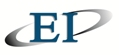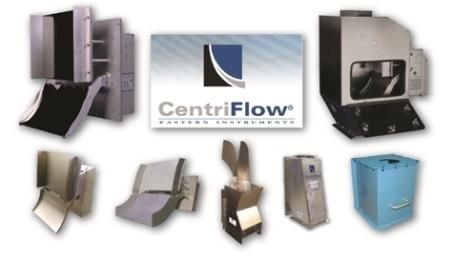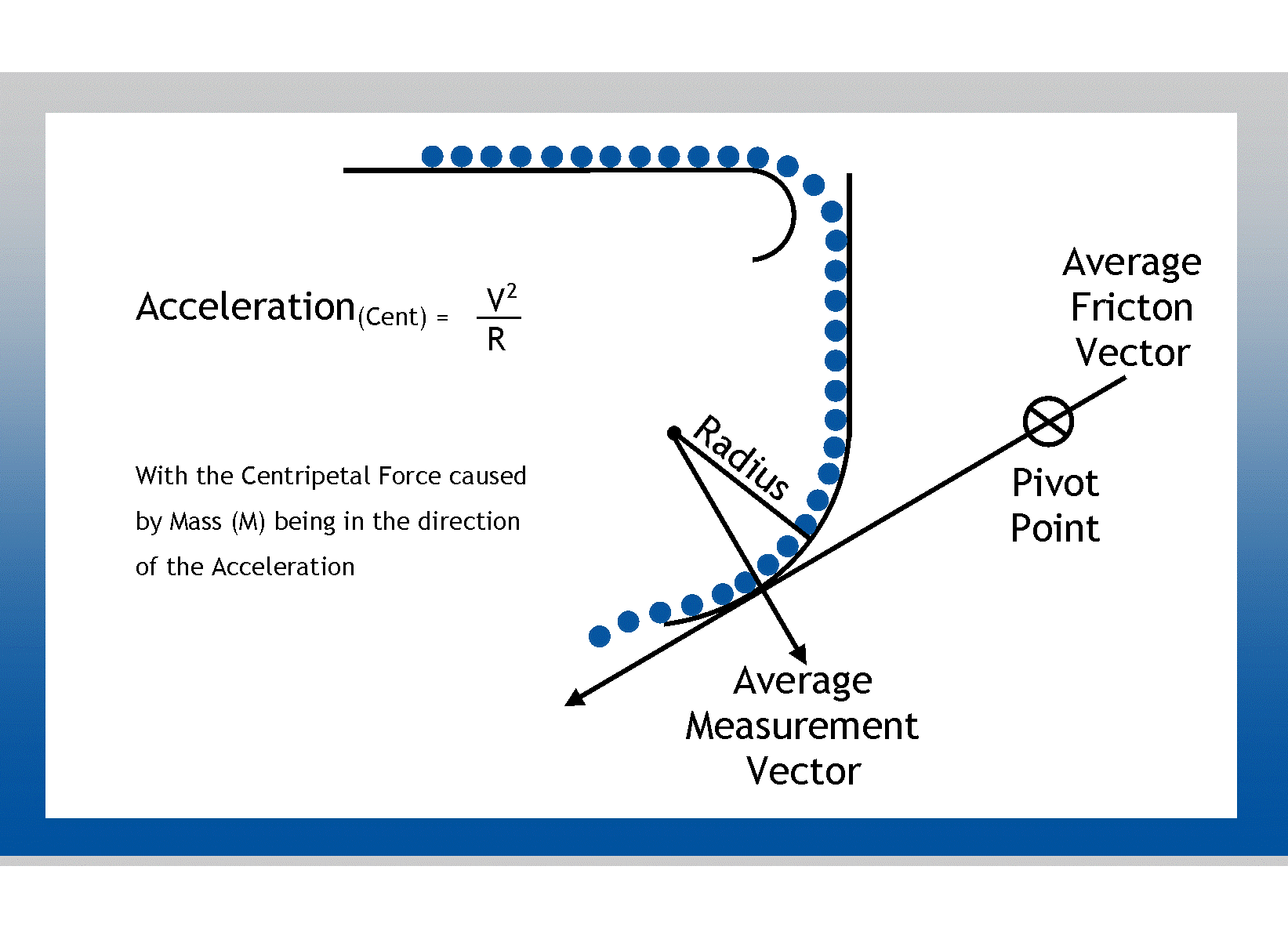EASTERN INSTRUMENTS

THE PRINCIPAL OF CENTRIPETAL FORCE AND THE SCIENCE BEHIND OUR SOLIDS FLOW MEASUREMENTThe CentriFlow® Meter from Eastern Instruments is a Solid Particle Mass Flow Meter that is durable, accurate, maintenance-free, compact, and cost-effective. It is unique and positioned to replace weigh belts, impact meters, loss in weight meters, and static weigh scales, anywhere that accuracy, low maintenance, and zero drift are paramount.

Below is a description of how Eastern Instruments has used the principal of centripetal force to accurately measure the flow of solids ranging from granular products that are extremely free flowing to the dustiest of powders.

CENTRIPETAL FORCE BASED FLOW MEASUREMENTTHE PRINCIPAL OF CENTRIPETAL FORCE AND EASTERN INSTRUMENTS

The secret to the amazing accuracy that can be achieved with Eastern Instruments' solids flow measurement products lies in their zero-friction patented design, which is based on the principle of centripetal force.

Centripetal force is the inward force required to keep an object moving in a circular path. It can be shown that an object moving in a circular path has acceleration toward the center of the circle along a radius. This radial acceleration, called the centripetal acceleration, is such that, if an object has a linear or tangential velocity when moving in a circular path of radius (R), the centripetal acceleration is V2/R. If the object undergoing the centripetal acceleration has a mass (M), then by Newton’s second law of motion, the centripetal force (Fc) is in the direction of acceleration. This is expressed by the formula:

Force = Mass x Velocity2